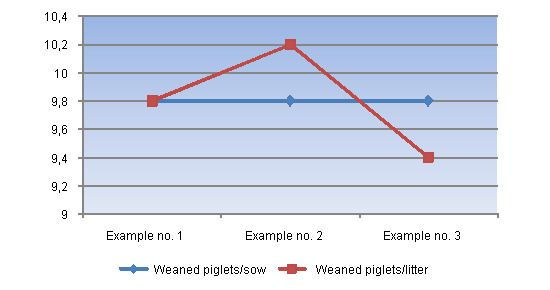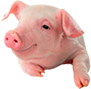I'm missing weaned piglets/sow: Why?

Normally, when we ask a farmer which is their average number of weaned piglets per sow they normally know this parameter, because its calculation is easy, nevertheless...

Monday 12 November 2012 (6 years 11 months 5 days ago)
Like

Normally, when we ask a farmer which is their average number of weaned piglets per sow they normally know this parameter, because its calculation is easy. The formula normally used is as follows:

- Average number of weaned piglets/sow during a certain period of time = Total number of piglets weaned during that period of time / Number of weaned sows during that period of time.

This formula is correct. Nevertheless, if we ask the following questions:

- How many sows have weaned 0 piglets during that period of time?
- How do these sows affect the average of weaned piglets/sow?
- How many nurse sows have been used during this period of time?
- How do these nurse sows affect the average of weaned piglets/sow?

Normally, the answer to these questions is not clear for the farmer. In order to evaluate the effect of these sows we should calculate another parameter: the average number of weaned piglets per litter. This datum will tell us the average of productive teats of the sows in our farm. In order to explain the calculation of this second parameter, we are going to take advantage of examples that are growingly more complicated but nearer to the real situation in the farms.

Example no. 1.- In a farm, during a month, 50 sows are weaned with a total of 490 weaned piglets. During this period of time, there are no sows that wean 0 piglets and no nurse sows are used.

In this case, the number of weaned piglets per sow is 490/50 = 9,8. Because the number of weaned sows and of weaned litters is the same, the average number of weaned piglets/litter is also 9,8.

Example no. 2.- On the following month the same farm also weans 50 sows and 490 piglets, but among the 50 weaned sows, 2 of them weaned 0 piglets (one of the sows died a few days after the farrowing and the piglets had to be taken away from the other one due to an infection that caused its drying off).

In this case the calculations would be:

- Weaned piglets/sow: It still is 490/50 (because there were 50 weaned sows), so it would be 9,8.

- Weaned piglets/litter: In this case it would be 490/48 (because there were two sows that did not get to wean piglets, so they did not wean any litter). This figure would be 10,2.

As it can be seen, the difference among both parameters is important.

Example no. 3.- This would be the example that reflects better the reality in the majority of the farms. The same farm weans 50 sows and 490 piglets, but two of those sows weaned 0 piglets and also, 40 of those piglets come from 4 nurse sows, this is, sows that weaned piglets during that month but that carried on nursing piglets and that were not weaned until the following month.

The mean number of weaned piglets/sow is still the same, but in order to know the number of weaned piglets/litter we must calculate the number of weaned litters during that period of time. In order to do this we must:

- Subtract from the 50 sows the two sows that weaned 0 piglets (because they did not wean any litter).

- Add to the 48 remaining sows the 4 nurse sows that were used during that period of time (because they represent weaned litters, but not weaned sows).

So, during that month we have 52 weaned sows. So, the average number of weaned piglets per litter is 490/52: this is, 9.4. Once more, the difference is considerable, but in this case, the number of weaned piglets/litter is lower than the number of weaned piglets/sow.

So, in order to be able to calculate both parameters it is only necessary to have the following data:

- Total number of weaned piglets during the period of time (N)

- Number of weaned sows during the period of time (A)

- Number of those weaned sows that did not wean any piglets (B)

- Number of nurse sows that were used during that period of time (C)

And the calculations are:

- Average number of weaned piglets/sow = N/A

- Average number of weaned piglets/litter = N/(A-B+C)As we can see in the Graph above, there are considerable differences among both parameters. So, it is convenient to obtain both results, because the datum weaned piglets/litter will tell us if the productivity that we are obtaining in the farrowing quarters is due to a good milk yield of the sows (a high number of weaned piglets/litter) or to an intensive use of the nurse sows because the sows (dams) do not get to wean many piglets (low number of weaned piglets/litter). We will also obtain information about the % of sows that suffer problems or die in the farrowing quarters (sows that wean 0 piglets).

Articles

Transfers of piglets until the 3rd-4th day after their birth16-Nov-2012 6 years 11 months 1 days ago
Nests for piglets in the farrowing quarters07-Nov-2012 6 years 11 months 10 days ago